# Overview of Kinematics Flashcards

Overview of Kinematics Flashcards
1/24 (missed) 0 0
Create Your Account To Continue Studying

As a member, you'll also get unlimited access to over 79,000 lessons in math, English, science, history, and more. Plus, get practice tests, quizzes, and personalized coaching to help you succeed.

Try it risk-free for 30 days. Cancel anytime
Acceleration

A vector quantity that represents how an object's velocity has changed over time

Equation: Δvelocity / Δtime = (vf - vi) / (tf - ti)

Got it
Find the average velocity for the trip.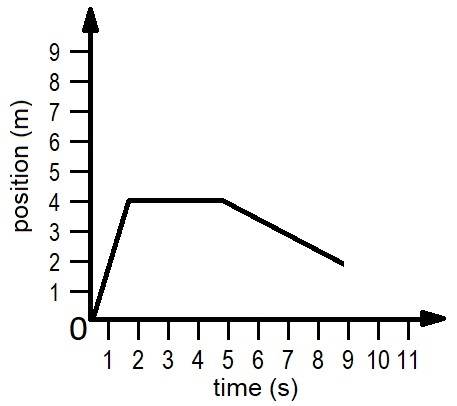Average velocity = change in position / change in time = (2 m - 0 m) / (9 s - 0 s) = 0.22 m/s

Got it
Find the instantaneous velocity at 7 s.

v = Δx / Δt = (2 m - 4 m) / (9 s - 5 s) = -0.5 m/s

Got it
Find the instantaneous velocity at 4 s.v = Δx / Δt = (4 m - 4 m) / (5 s - 2 s) = 0 m/s

Got it
Describe the motion in this graph.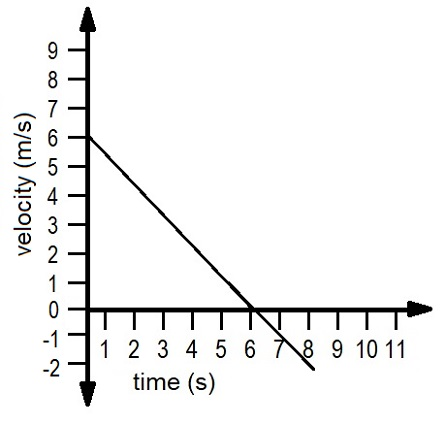The object is slowing down from 0-6 seconds, is not moving at 6 seconds, then accelerating in the opposite direction starting after 6 seconds.

Got it
Find the velocity.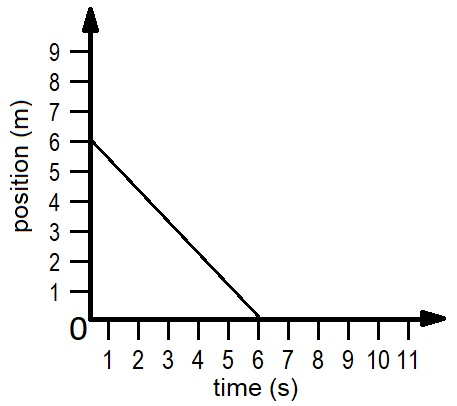Δs / Δt = -6 m / 6 s = -1 m/s

Got it
Velocity

A vector quantity that describes the total displacement traveled over a period of time

Change in position (displacement) / change in time

Got it
Speed

A scalar quantity that describes the total distance traveled over a period of time

Got it
Displacement

A vector quantity that represents the shortest distance between the starting and ending point of a path taken by an object

Got it
Distance

A scalar quantity that represents the total length of the path taken by an object between two points

Got it
Identify what occurs at the circle.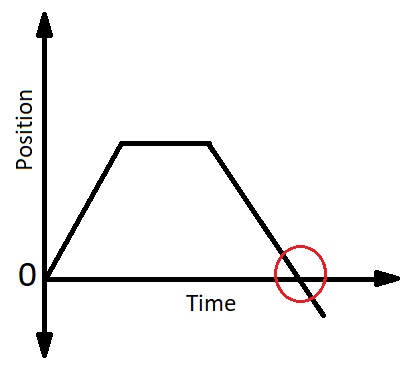The object is back at the starting position

Got it
Position

A location in space, identified by a number on the x- and/or y-axis

Can be a positive or negative value

Got it

or choose a specific lesson: See all lessons in this chapter
24 cards in set

## Flashcard Content Overview

One of the most important things you will learn in physics is how to describe motion. These flashcards will help you do just that. They will review the definitions of words used to describe motion, like velocity and acceleration, and will help you practice solving problems involving motion using common kinematics equations.

Front
Back
Position

A location in space, identified by a number on the x- and/or y-axis

Can be a positive or negative value

Identify what occurs at the circle.The object is back at the starting position

Distance

A scalar quantity that represents the total length of the path taken by an object between two points

Displacement

A vector quantity that represents the shortest distance between the starting and ending point of a path taken by an object

Speed

A scalar quantity that describes the total distance traveled over a period of time

Velocity

A vector quantity that describes the total displacement traveled over a period of time

Change in position (displacement) / change in time

Find the velocity.Δs / Δt = -6 m / 6 s = -1 m/s

Describe the motion in this graph.The object is slowing down from 0-6 seconds, is not moving at 6 seconds, then accelerating in the opposite direction starting after 6 seconds.

Find the instantaneous velocity at 4 s.v = Δx / Δt = (4 m - 4 m) / (5 s - 2 s) = 0 m/s

Find the instantaneous velocity at 7 s.

v = Δx / Δt = (2 m - 4 m) / (9 s - 5 s) = -0.5 m/s

Find the average velocity for the trip.Average velocity = change in position / change in time = (2 m - 0 m) / (9 s - 0 s) = 0.22 m/s

Acceleration

A vector quantity that represents how an object's velocity has changed over time

Equation: Δvelocity / Δtime = (vf - vi) / (tf - ti)

Find the acceleration.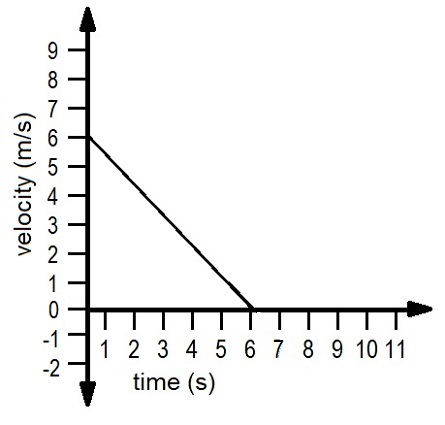Acceleration = (final velocity - initial velocity) / (final time - starting time) = (0 m/s - 6 m/s) / (6 s - 0 s) = -1 m/s2

Negative Acceleration

Indicates that the velocity is decreasing over time, meaning it is slowing down

Also called deceleration

Find the angular acceleration of an object that has sped up from 15 radians/s over 10 seconds to 65 radians/s.

v = v0 + at

A car accelerates from 25 m/s over 30 seconds, covering 900 meters. Find the rate of acceleration.

x = x0 + v0 * t + 1/2 * a * t2

900 m = 0 + (25 m/s * 30 s) + 1/2 * a * (30 s)2

a = 0.33 m/s2

Find the final velocity of a cat that accelerates at a rate of 0.5 m/s2 over 40 meters with a starting velocity of 2 m/s.

v2 = v02 + 2 * a * (x - x0)

v2 = (2 m/s)2 + 2 * 0.5 m/s2 * (40 m - 0 m)

v = 6.6 m/s

Find the initial velocity of a car that has accelerated 1 m/s2 over 10 seconds to a final velocity of 35 m/s.

v = v0 + at

35 m/s = v0 + 1 m/s2 * 10 s

v0 = 25 m/s

Find the distance covered by a cat that accelerates at a rate of 0.5 m/s2 from rest to 5 m/s.

v2 = v02 + 2 * a * (x - x0)

(5 m/s)2 = (0 m/s)2 + 2 * 0.5 m/s2 * (x - 0 m)

x = 25 m

Projectile Motion

A parabolic path traveled by a projectile launched at a specific speed and angle whose vertical acceleration is only determined by acceleration of gravity (a = g = 9.8 m/s2 downward)

Find the vertical and horizontal velocity of a projectile at the top of its arc that was released at a velocity of 35 m/s north at 60 degrees above the horizontal.

Vertical component of velocity at top of arc is always equal to 0 m/s, regardless of initial velocity

Horizontal component of velocity is constant, with v = 35 m/s * cos(60°) = 17.5 m/s north

Find the initial upward velocity required to get a cannonball over a 10 meter wall that is 20 meters away.

vfy2 = viy2 + 2 * ay * y

y = 10 m, viy = 0 m/s

0 = viy2 + 2 * -9.8 m/s2 * 10 m = 196 m2/s2

viy = 14 m/s

Describe the object's distance and direction.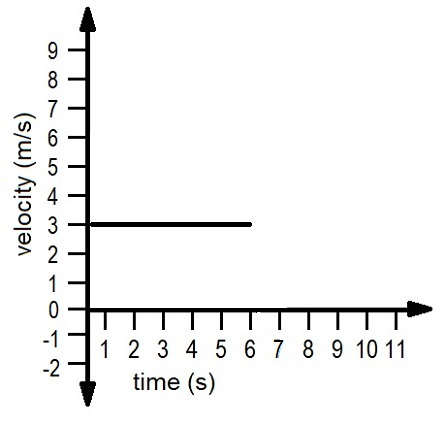The object is moving at a rate of 3 m/s for 6 seconds. 3 m/s * 6 s = 18 meters. Since the velocity is positive, the object is moving away from us.

Describe what happens at 1, 2, and 3.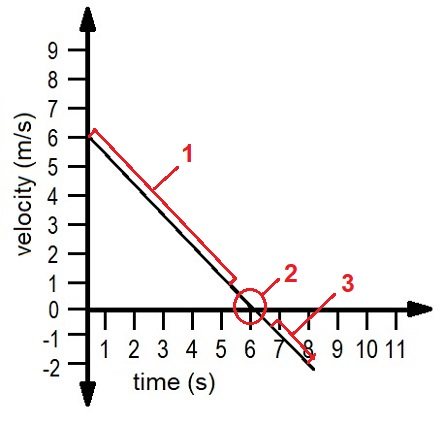1. The object has constant negative acceleration in one direction.

2. The object stops and turns around at 6 s.

3. The object has constant positive acceleration in the opposite direction.

To unlock this flashcard set you must be a Study.com Member.